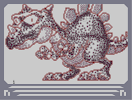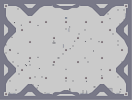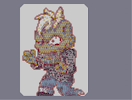### DrakeHover over the thumbnail for a full-size version.

Author SilentDemon author:silentdemon bitesized n-art rated 2006-06-10 4 by 40 people. \$Drake#SilentDemon#none#500000000000000000021PO00000000000000000000E1O00000000000000000000PE100000000000000000000PPE00000000000000000000PPP00000000000000000000PPP00000000000000000000PPP00000000000000000000PPP00000000000000000000PPP00000000000000000000PPP00000000000000000000PPP00000000000000000000PPP00000000000000000000PPP00000000000000000000PPP00000000000000000000PPP00000000000000000000PPP00000000000000000000PPP00000000000000000000PPP00000000000000000000PPP00000000000000000000PPP00000000000000000000PPP00000000000000000000PPP00000000000000000000PPP00000000000000000000PPP00000000000000000000PPP00000000000000000000PPP00000000000000000000PPP00000000000000000000PPD00000000000000000000PD100000000000000000000DPO4000000000000000000311O|5^84,492!12^42,222!12^42,228!12^48,216!12^54,216!12^54,210!12^54,204!12^54,198!12^60,192!12^60,186!12^54,180!12^54,174!12^48,168!12^48,162!12^48,156!12^54,150!12^60,150!12^66,156!12^72,156!12^78,162!12^84,162!12^90,162!12^96,162!12^102,162!12^108,162!12^90,168!12^96,174!12^96,180!12^96,186!12^90,192!12^66,198!12^72,198!12^78,198!12^84,198!12^78,150!12^78,144!12^84,138!12^84,132!12^84,126!12^84,114!12^84,108!12^90,102!12^96,102!12^102,102!12^90,138!12^96,144!12^102,150!12^108,150!12^114,144!12^120,138!12^126,132!12^126,126!12^126,120!12^120,114!12^114,108!12^108,108!12^96,126!12^102,132!12^96,120!12^78,120!12^72,114!12^72,102!12^72,108!12^72,96!12^78,90!12^84,90!12^96,84!12^90,84!12^102,84!12^108,84!12^114,84!12^120,84!12^114,78!12^120,72!12^126,72!12^132,72!12^138,72!12^132,66!12^132,60!12^138,54!12^138,48!12^138,42!12^138,36!12^138,30!12^144,54!12^144,24!12^150,24!12^156,30!12^162,36!12^168,42!12^150,48!12^156,48!12^162,48!12^174,48!12^180,48!12^186,54!12^192,60!12^198,60!12^204,60!12^210,60!12^216,60!12^222,60!12^192,66!12^186,66!12^180,72!12^174,72!12^228,66!12^234,66!12^240,66!12^246,66!12^240,72!12^246,78!12^132,126!12^132,120!12^132,114!12^132,108!12^132,102!12^138,120!12^138,126!12^144,114!12^150,108!12^156,102!12^162,96!12^168,96!12^174,90!12^180,90!12^186,90!12^192,84!12^198,84!12^204,84!12^210,84!12^216,90!12^222,90!12^228,96!12^234,96!12^240,102!12^246,102!12^252,102!12^258,96!12^144,132!12^150,138!12^156,144!12^162,144!12^168,150!12^174,150!12^180,150!12^186,150!12^192,150!12^198,144!12^204,144!12^210,144!12^216,138!12^222,138!12^228,138!12^222,132!12^222,126!12^234,132!12^228,120!12^228,114!12^234,108!12^192,126!12^192,120!12^192,114!12^114,168!12^120,168!12^126,174!12^126,180!12^60,222!12^66,228!12^72,228!12^78,228!12^120,204!12^114,210!12^36,234!12^36,240!12^36,246!12^36,252!12^36,258!12^36,264!12^42,270!12^42,276!12^48,282!12^54,288!12^60,294!12^66,294!12^72,294!12^78,288!12^84,282!12^90,282!12^90,276!12^96,276!12^102,276!12^108,270!12^114,270!12^120,270!12^126,264!12^132,264!12^138,258!12^144,252!12^150,246!12^156,240!12^162,240!12^168,234!12^174,234!12^180,234!12^186,234!12^192,234!12^198,234!12^96,288!12^102,288!12^108,288!12^114,294!12^120,294!12^126,294!12^132,294!12^138,294!12^144,294!12^150,288!12^156,288!12^162,288!12^168,288!12^252,60!12^204,228!12^210,228!12^216,222!12^222,216!12^228,210!12^174,282!12^180,282!12^186,276!12^192,270!12^198,264!12^204,264!12^210,258!12^216,252!12^222,252!12^228,252!12^234,258!12^240,258!12^246,258!12^252,258!12^258,252!12^264,252!12^270,252!12^276,246!12^282,246!12^288,240!12^294,234!12^300,228!12^300,222!12^306,216!12^306,210!12^306,204!12^312,198!12^312,192!12^312,186!12^312,180!12^312,174!12^234,198!12^240,186!12^240,180!12^234,204!12^240,192!12^246,174!12^246,168!12^252,162!12^258,156!12^264,156!12^270,156!12^276,156!12^282,156!12^288,156!12^294,162!12^300,162!12^306,168!12^318,174!12^258,54!12^264,54!12^270,54!12^276,54!12^276,48!12^270,48!12^282,42!12^288,36!12^294,36!12^300,30!12^306,24!12^312,24!12^318,30!12^318,36!12^318,42!12^312,48!12^312,54!12^312,60!12^306,66!12^306,72!12^306,78!12^300,72!12^294,66!12^288,60!12^282,60!12^312,84!12^312,90!12^312,96!12^312,102!12^306,96!12^300,90!12^294,84!12^288,84!12^324,180!12^330,180!12^336,174!12^336,168!12^330,168!12^330,162!12^324,156!12^318,150!12^318,144!12^318,108!12^318,114!12^324,120!12^324,126!12^330,132!12^336,138!12^342,144!12^348,150!12^354,156!12^360,162!12^366,168!12^372,168!12^372,174!12^378,174!12^384,174!12^390,174!12^384,180!12^390,186!12^390,192!12^396,198!12^396,204!12^396,210!12^396,222!12^396,216!12^300,240!12^300,246!12^300,252!12^306,258!12^312,264!12^312,270!12^318,276!12^318,282!12^318,288!12^312,282!12^306,288!12^306,294!12^306,300!12^306,306!12^306,312!12^312,318!12^300,306!12^294,300!12^288,294!12^282,288!12^276,282!12^270,276!12^264,276!12^258,276!12^252,276!12^246,282!12^240,288!12^240,294!12^240,300!12^240,306!12^234,306!12^228,306!12^222,312!12^216,318!12^216,324!12^216,330!12^222,336!12^228,342!12^246,312!12^252,318!12^258,318!12^258,324!12^264,312!12^270,318!12^270,324!12^270,330!12^264,330!12^276,312!12^276,306!12^288,324!12^282,330!12^276,342!12^276,336!12^282,342!12^234,348!12^240,348!12^240,342!12^246,342!12^252,342!12^258,342!12^258,336!12^246,354!12^252,354!12^252,348!12^288,348!12^270,348!12^264,348!12^270,354!12^276,354!12^282,354!12^288,354!12^294,354!12^300,348!12^306,342!12^306,336!12^306,330!12^300,324!12^312,348!12^318,354!12^324,360!12^330,360!12^336,360!12^342,354!12^348,348!12^324,294!12^324,300!12^330,306!12^336,312!12^336,318!12^342,324!12^348,324!12^348,318!12^348,330!12^354,342!12^354,336!12^354,330!12^354,312!12^360,312!12^366,312!12^366,306!12^366,300!12^372,318!12^378,324!12^378,330!12^384,324!12^360,342!12^360,336!12^366,336!12^372,336!12^378,336!12^384,336!12^390,336!12^390,330!12^396,330!12^366,348!12^372,354!12^378,360!12^384,366!12^390,372!12^396,378!12^402,378!12^408,384!12^414,384!12^396,180!12^402,180!12^408,186!12^414,192!12^390,168!12^396,168!12^402,168!12^408,162!12^408,156!12^408,150!12^402,144!12^396,144!12^384,162!12^378,162!12^372,156!12^390,138!12^366,150!12^366,144!12^366,138!12^366,132!12^372,132!12^378,132!12^384,132!12^402,138!12^402,132!12^402,126!12^408,132!12^414,138!12^420,144!12^426,144!12^396,120!12^390,114!12^384,108!12^384,102!12^384,96!12^384,90!12^384,84!12^390,78!12^390,72!12^390,66!12^396,60!12^396,54!12^396,48!12^402,48!12^408,48!12^414,48!12^420,54!12^426,54!12^432,54!12^438,54!12^444,54!12^450,60!12^450,66!12^450,72!12^456,78!12^456,84!12^456,90!12^462,84!12^468,78!12^432,138!12^438,132!12^444,126!12^450,120!12^456,114!12^462,108!12^462,102!12^462,96!12^360,294!12^366,288!12^372,294!12^372,300!12^378,288!12^378,294!12^378,300!12^378,306!12^354,288!12^348,282!12^348,276!12^348,270!12^348,264!12^354,258!12^360,252!12^366,252!12^372,252!12^378,258!12^378,264!12^384,270!12^384,276!12^384,282!12^390,270!12^396,264!12^402,264!12^408,264!12^408,258!12^414,270!12^414,276!12^414,282!12^414,288!12^420,276!12^408,294!12^402,300!12^396,306!12^384,306!12^384,312!12^390,312!12^390,300!12^402,336!12^402,342!12^408,348!12^414,348!12^420,354!12^426,354!12^432,354!12^438,348!12^444,348!12^402,252!12^402,246!12^396,240!12^390,234!12^384,234!12^378,228!12^372,228!12^372,222!12^372,216!12^366,210!12^360,204!12^354,198!12^348,192!12^342,192!12^336,186!12^414,300!12^414,306!12^414,312!12^414,318!12^420,306!12^426,312!12^432,312!12^438,318!12^438,324!12^420,390!12^426,390!12^432,390!12^438,390!12^444,390!12^444,396!12^450,396!12^456,396!12^450,402!12^468,402!12^462,402!12^474,402!12^480,402!12^486,402!12^492,402!12^498,402!12^504,402!12^456,408!12^462,414!12^468,414!12^474,414!12^480,420!12^480,426!12^474,432!12^474,438!12^468,444!12^462,444!12^456,450!12^450,456!12^444,462!12^438,462!12^432,468!12^426,468!12^420,468!12^414,474!12^408,480!12^396,480!12^402,480!12^390,480!12^384,480!12^378,480!12^372,480!12^366,480!12^360,480!12^354,480!12^348,480!12^342,480!12^336,480!12^330,480!12^324,480!12^318,480!12^312,480!12^306,480!12^300,480!12^294,480!12^288,480!12^282,480!12^276,480!12^270,480!12^264,480!12^258,480!12^378,474!12^372,468!12^366,468!12^360,474!12^366,462!12^372,456!12^378,450!12^378,444!12^372,444!12^366,438!12^360,438!12^354,438!12^348,438!12^342,438!12^336,438!12^330,444!12^324,444!12^318,444!12^324,450!12^312,438!12^306,438!12^300,438!12^294,438!12^288,444!12^282,444!12^276,450!12^270,456!12^270,462!12^276,462!12^264,468!12^258,474!12^318,456!12^318,462!12^324,462!12^312,468!12^306,474!12^330,468!12^336,474!12^282,468!12^288,474!12^384,438!12^390,438!12^396,438!12^402,432!12^408,432!12^414,426!12^420,426!12^426,426!12^420,420!12^426,420!12^432,420!12^438,420!12^420,414!12^420,408!12^414,402!12^408,402!12^402,402!12^396,396!12^390,396!12^384,390!12^378,384!12^378,378!12^378,372!12^510,396!12^516,396!12^522,396!12^528,390!12^534,390!12^540,390!12^546,384!12^552,384!12^558,378!12^564,378!12^564,372!12^558,372!12^552,372!12^546,372!12^540,372!12^534,372!12^528,366!12^522,366!12^516,366!12^570,372!12^576,372!12^588,372!12^582,372!12^594,372!12^510,360!12^504,354!12^504,348!12^498,342!12^498,336!12^498,330!12^498,324!12^504,318!12^504,312!12^510,306!12^510,300!12^516,294!12^516,288!12^600,378!12^606,378!12^612,378!12^618,378!12^624,384!12^630,384!12^636,390!12^630,396!12^624,402!12^618,408!12^612,414!12^606,414!12^600,420!12^594,420!12^588,420!12^582,420!12^576,420!12^570,420!12^564,420!12^558,426!12^552,426!12^546,426!12^540,432!12^534,438!12^534,444!12^528,438!12^522,432!12^516,432!12^510,432!12^504,432!12^498,438!12^492,438!12^486,444!12^480,450!12^480,456!12^480,462!12^486,456!12^492,456!12^474,468!12^468,474!12^468,480!12^498,462!12^504,468!12^504,474!12^474,480!12^480,480!12^486,480!12^492,480!12^498,480!12^504,480!12^510,480!12^516,480!12^522,480!12^528,480!12^534,480!12^540,480!12^546,480!12^552,480!12^558,480!12^564,480!12^570,480!12^576,480!12^582,480!12^528,450!12^528,456!12^528,462!12^528,468!12^534,474!12^540,468!12^540,462!12^546,456!12^552,450!12^558,450!12^564,456!12^570,462!12^576,468!12^576,474!12^588,480!12^594,474!12^594,468!12^594,462!12^594,456!12^594,450!12^600,444!12^606,438!12^600,468!12^600,474!12^600,480!12^606,462!12^612,456!12^618,456!12^624,456!12^630,462!12^636,468!12^636,474!12^630,480!12^624,480!12^636,480!12^642,480!12^648,480!12^618,480!12^612,480!12^606,480!12^654,474!12^660,468!12^660,462!12^660,456!12^660,450!12^654,444!12^648,438!12^648,432!12^648,426!12^654,420!12^654,414!12^654,408!12^660,402!12^660,396!12^666,390!12^672,384!12^672,378!12^672,372!12^672,366!12^666,366!12^660,366!12^654,366!12^660,372!12^648,360!12^642,354!12^636,348!12^630,348!12^624,348!12^618,348!12^612,348!12^606,348!12^600,348!12^624,342!12^630,336!12^630,330!12^636,324!12^642,324!12^648,324!12^654,324!12^660,324!12^666,324!12^672,324!12^678,324!12^684,324!12^690,318!12^702,318!12^696,318!12^708,312!12^714,312!12^720,312!12^726,306!12^732,306!12^738,300!12^744,294!12^750,288!12^756,282!12^762,276!12^756,276!12^750,276!12^744,276!12^762,270!12^768,270!12^768,264!12^738,282!12^732,282!12^726,288!12^720,282!12^714,282!12^708,282!12^702,282!12^696,288!12^690,288!12^684,282!12^678,276!12^672,276!12^666,276!12^660,276!12^654,276!12^654,270!12^654,282!12^654,288!12^648,294!12^648,300!12^648,306!12^642,318!12^642,312!12^516,276!12^516,282!12^516,270!12^516,264!12^522,258!12^522,252!12^522,246!12^528,240!12^522,282!12^510,288!12^504,282!12^498,282!12^492,282!12^492,276!12^492,270!12^528,276!12^528,270!12^534,264!12^540,258!12^486,288!12^486,294!12^426,300!12^426,294!12^432,288!12^432,282!12^426,270!12^432,270!12^438,276!12^444,270!12^444,264!12^450,342!12^456,336!12^462,330!12^468,324!12^468,318!12^474,312!12^474,306!12^474,300!12^474,294!12^474,288!12^480,300!12^474,72!12^474,78!12^474,84!12^474,90!12^468,66!12^468,60!12^468,54!12^468,48!12^468,42!12^468,36!12^468,30!12^474,24!12^480,96!12^486,90!12^492,90!12^498,84!12^504,84!12^510,78!12^516,78!12^522,78!12^522,72!12^522,66!12^528,60!12^528,54!12^528,48!12^534,42!12^534,36!12^534,30!12^534,24!12^534,60!12^540,60!12^546,54!12^546,60!12^546,66!12^540,72!12^546,72!12^552,72!12^552,48!12^552,42!12^552,36!12^558,30!12^558,24!12^558,78!12^564,78!12^570,78!12^576,84!12^582,90!12^588,90!12^588,84!12^594,78!12^600,72!12^606,66!12^612,60!12^612,54!12^612,48!12^612,42!12^612,36!12^612,30!12^612,24!12^594,96!12^600,96!12^600,102!12^600,108!12^606,90!12^612,84!12^618,78!12^624,78!12^630,78!12^636,78!12^642,84!12^648,84!12^654,90!12^654,102!12^654,96!12^654,108!12^654,114!12^606,114!12^606,120!12^612,126!12^612,132!12^618,132!12^624,132!12^648,120!12^642,126!12^636,126!12^630,126!12^618,138!12^618,144!12^618,150!12^630,150!12^624,150!12^624,156!12^624,162!12^624,168!12^636,144!12^642,144!12^648,144!12^654,144!12^660,150!12^666,156!12^672,162!12^666,168!12^660,174!12^630,168!12^630,174!12^630,180!12^630,186!12^636,186!12^642,186!12^654,180!12^648,186!12^636,192!12^636,198!12^636,204!12^642,204!12^642,210!12^642,216!12^648,222!12^648,228!12^654,234!12^660,228!12^666,222!12^672,216!12^672,210!12^672,204!12^672,198!12^666,198!12^660,198!12^654,198!12^648,198!12^660,240!12^666,240!12^672,240!12^678,240!12^678,246!12^684,246!12^690,252!12^696,252!12^702,252!12^708,252!12^708,246!12^708,234!12^708,240!12^708,228!12^702,222!12^696,222!12^690,222!12^684,234!12^684,228!12^714,246!12^720,246!12^732,246!12^726,246!12^726,240!12^726,234!12^726,228!12^726,222!12^732,216!12^732,210!12^738,210!12^744,216!12^738,240!12^744,240!12^750,222!12^750,228!12^750,234!12^756,216!12^756,210!12^756,204!12^756,198!12^756,192!12^762,216!12^768,210!12^762,192!12^768,NaN!12^768,198!3^766,205!3^742,227!3^742,237!3^734,239!3^701,237!3^699,246!3^690,246!3^663,216!3^656,226!3^651,216!3^650,206!3^655,169!3^646,159!3^635,159!3^640,170!3^642,181!3^640,97!3^640,109!3^631,119!3^618,124!3^620,113!3^628,105!3^626,93!3^614,99!3^610,109!3^581,79!3^588,69!3^595,62!3^646,115!3^651,103!3^650,95!3^605,56!3^605,44!3^604,29!3^596,35!3^589,44!3^592,54!3^580,56!3^575,68!3^563,66!3^569,54!3^570,43!3^578,30!3^589,28!3^581,41!3^514,69!3^518,57!3^520,45!3^525,35!3^514,31!3^509,43!3^506,53!3^503,63!3^500,76!3^488,80!3^488,69!3^494,53!3^497,43!3^501,32!3^488,32!3^487,44!3^488,57!3^525,27!3^441,62!3^441,72!3^446,82!3^448,96!3^455,104!3^447,114!3^436,100!3^433,87!3^446,105!3^439,92!3^430,76!3^430,68!3^421,62!3^428,57!3^437,111!3^437,120!3^428,128!3^419,122!3^427,115!3^426,102!3^421,91!3^419,81!3^415,70!3^413,60!3^407,74!3^402,85!3^401,94!3^401,103!3^414,92!3^416,101!3^413,109!3^383,142!3^390,152!3^400,157!2^650,219,0.707106781186547,-0.707106781186547!2^643,179,0,-1!2^655,176,-0.707106781186547,-0.707106781186547!2^635,122,0,-1!2^624,125,-0.707106781186547,-0.707106781186547!2^646,116,-0.707106781186547,-0.707106781186547!2^648,108,-1,0!2^586,79,-0.707106781186547,-0.707106781186547!2^595,68,-0.707106781186547,-0.707106781186547!2^604,62,-0.707106781186547,-0.707106781186547!2^605,51,-1,0!2^605,41,-1,0!2^488,83,-0.707106781186547,-0.707106781186547!2^499,80,0,-1!2^508,73,0,-1!2^513,66,-0.707106781186547,-0.707106781186547!2^520,59,-1,0!2^522,46,-1,0!2^529,40,-1,0!2^527,31,-1,0!2^444,64,-1,0!2^446,79,-1,0!2^451,85,-1,0!2^456,99,-1,0!2^447,111,-0.707106781186547,-0.707106781186547!2^442,124,-0.707106781186547,-0.707106781186547!2^452,114,-0.707106781186547,-0.707106781186547!2^430,129,-0.707106781186547,-0.707106781186547!2^405,157,-1,0!3^74,175!3^85,177!3^79,187!3^311,41!3^305,51!3^298,61!3^155,43!3^156,40!3^158,42!3^302,61!3^304,57!3^309,49!3^300,69!3^304,69!12^498,360!12^492,366!12^486,372!12^480,378!12^474,378!12^468,378!12^462,384!12^456,384!12^450,384!12^312,216!12^318,210!12^324,210!12^330,204!12^336,198!12^336,192!12^366,234!12^360,240!12^354,246!12^348,252!12^342,252!12^336,258!12^330,258!12^324,258!12^318,258!12^312,258!12^336,306!12^342,306!12^348,300!12^690,294!12^690,312!12^696,306!12^696,300!12^732,294!12^732,300!3^389,308!3^369,296!3^383,333!3^279,352!3^262,323!3^247,347!3^625,468!3^625,470!3^621,472!3^622,475!3^630,475!3^609,471!3^614,464!3^613,468!3^542,474!3^542,469!3^544,464!3^550,458!3^566,466!3^561,470!3^561,473!3^569,471!3^555,466!3^605,473!3^632,476!3^497,472!3^491,475!3^488,475!3^494,475!3^497,473!3^484,461!3^472,473!3^372,471!3^372,474!3^330,478!3^327,478!3^317,471!3^314,473!3^276,470!3^274,474!3^279,474!3^112,279!3^121,273!3^98,281!3^132,266!3^141,259!3^148,270!3^139,275!3^128,282!3^120,285!3^139,286!3^157,278!3^158,260!3^173,271!3^185,238!3^199,241!3^208,236!3^217,232!3^224,222!3^224,218!3^233,246!3^244,247!3^254,245!3^265,240!3^279,236!3^287,230!3^293,217!3^296,174!3^301,184!3^285,167!3^261,162!3^254,168!3^252,177!3^498,295!3^490,303!3^483,312!3^495,313!3^502,302!3^493,325!3^492,335!3^481,340!3^472,329!3^477,320!3^492,347!3^490,356!3^480,356!3^471,343!3^462,343!3^463,352!3^472,359!3^481,365!3^470,371!3^459,365!3^450,351!3^447,362!3^451,374!3^459,375!3^441,380!3^440,371!3^436,359!3^427,367!3^417,360!3^430,376!3^423,384!3^414,373!3^401,374!3^404,364!3^407,355!3^394,357!3^391,367!3^382,353!3^373,345!3^391,345!3^505,369!3^496,377!3^486,385!3^474,387!3^458,391!3^467,396!3^480,396!3^497,393!3^506,384!3^515,375!3^525,376!3^538,382!3^550,380!3^519,388!3^426,278!3^422,288!3^418,298!3^366,246!3^376,239!3^388,245!3^395,256!3^386,262!3^344,266!3^339,279!3^329,269!3^321,266!3^328,279!3^330,290!3^335,301!3^343,290!3^344,312!3^355,305!3^349,296!3^355,203!3^359,211!3^362,222!3^328,219!3^340,234!3^334,251!3^322,240!3^314,229!3^308,240!3^320,250!3^311,253!3^295,197!3^284,185!3^278,199!3^272,176!3^273,162!3^276,219!3^259,226!3^243,234!3^200,254!3^220,245!2^661,287,1,0!2^661,292,1,0!2^658,291,1,0!2^656,297,1,0!2^653,304,1,0!2^653,303,1,0!2^651,316,0.707106781186547,0.707106781186547!2^653,313,0.707106781186547,0.707106781186547!2^651,310,0.707106781186547,0.707106781186547!3^669,284!3^670,292!3^666,302!3^662,313!3^674,317!3^675,308!3^682,297!3^680,287!3^689,303!3^686,312!3^701,295!3^709,289!3^720,295!3^725,304!3^710,301!3^701,309!3^715,309!3^736,290!3^747,283!3^744,295!10^614,147!10^614,154!10^616,165!10^619,171!10^622,179!10^622,186!10^617,163!10^615,163!10^624,190!10^629,193!10^630,200!10^623,203!10^628,210!10^624,196!10^625,211!10^633,215!10^637,224!10^643,232!10^647,238!10^660,250!10^654,244!10^664,248!10^672,248!10^675,273!10^665,270!10^660,266!10^654,256!10^647,256!10^647,248!10^642,246!10^639,240!10^635,235!10^630,230!10^625,222!10^621,223!10^610,225!10^612,224!10^619,231!10^621,214!10^626,237!10^603,219!10^640,259!10^646,264!10^639,268!10^645,273!10^645,280!10^636,277!10^641,288!10^647,291!10^636,297!10^640,300!10^634,306!10^638,311!10^631,315!10^608,343!10^616,342!10^623,338!10^625,330!10^629,320!10^404,390!10^407,396!10^412,392!10^418,396!10^428,396!10^434,399!10^438,402!10^433,408!10^425,403!10^603,138!10^597,124!10^590,110!10^577,120!10^574,135!10^589,137!10^573,151!10^579,162!10^584,174!10^581,191!10^572,204!10^595,184!10^553,212!10^542,214!10^528,220!10^514,224!10^502,232!10^493,240!10^483,255!10^474,269!10^465,284!10^462,298!10^458,310!10^452,325!10^537,268!10^529,281!10^521,296!10^513,314!10^509,332!10^514,352!10^550,253!10^554,237!10^561,223!10^591,150!10^600,162!10^606,182!10^596,172!10^610,197!10^602,208!10^596,199!10^617,209!10^498,261!10^481,281!10^506,274!10^511,259!10^502,251!10^516,241!10^529,232!10^542,227!10^541,239!10^533,251!10^524,265!10^486,269!10^508,240!10^603,150!10^611,175!10^588,159!10^587,125!10^580,109!10^585,96!10^564,213!10^583,201!10^597,212!10^579,145!10^586,213!10^574,218!10^591,224!10^583,226!10^598,232!10^609,237!10^622,245!10^610,248!10^633,251!10^626,260!10^616,259!10^624,272!10^603,259!10^569,237!10^587,249!10^561,263!10^547,287!10^536,313!10^535,341!10^536,360!10^552,362!10^563,362!10^593,368!10^599,368!10^601,372!10^607,372!10^611,374!10^615,376!10^622,378!10^625,383!10^664,375!10^664,380!10^662,386!10^655,398!10^648,409!10^643,418!10^642,428!10^642,435!10^650,441!10^648,437!10^644,429!10^643,418!10^648,405!10^660,387!10^658,394!10^594,440!10^586,443!10^585,453!10^586,465!10^589,470!10^522,471!10^521,463!10^521,451!10^605,422!10^614,423!10^624,412!10^628,401!10^646,448!10^654,448!10^653,453!10^608,280!10^613,270!10^619,282!10^628,287!10^622,293!10^611,290!10^599,270!10^598,283!10^599,295!10^614,301!10^624,308!10^614,315!10^603,308!10^606,322!10^612,322!10^604,334!10^614,331!10^595,330!10^595,315!10^591,304!10^585,289!10^579,304!10^574,319!10^563,331!10^553,344!10^569,347!10^582,334!10^587,322!10^582,348!10^583,361!10^599,355!10^561,316!10^568,299!10^586,272!10^526,352!10^521,338!10^521,322!10^543,352!10^571,360!10^591,343!10^589,356!10^559,354!10^571,336!10^612,367!10^620,370!10^623,372!10^625,377!10^630,379!10^630,385!10^611,355!10^621,357!10^627,361!10^637,361!10^634,370!10^646,373!10^651,385!10^640,385!10^643,394!10^635,409!10^627,427!10^617,437!10^605,455!10^629,445!10^643,464!10^649,473!10^586,427!10^573,435!10^555,433!10^568,451!10^546,446!10^536,460!10^506,456!10^490,451!10^507,442!10^509,477!1^507,117!1^517,104!1^528,103!1^270,136!1^280,136!10^432,410!10^437,411!10^442,409!10^389,383!10^473,423!10^467,434!10^459,439!10^450,443!10^442,448!10^433,453!10^441,457!10^426,460!10^416,462!10^410,468!10^403,473!10^448,427!10^460,423!10^453,433!10^445,441!10^433,451!10^429,452!10^422,455!10^414,461!10^410,465!10^458,438!10^468,429!10^468,425!10^451,418!10^433,426!10^433,436!10^419,434!10^410,447!10^422,445!10^401,442!10^388,450!10^314,452!10^309,458!10^308,462!10^307,466!10^370,448!10^368,448!10^360,452!10^358,457!10^386,463!10^401,462!10^345,470!10^336,457!10^347,447!10^297,446!10^285,458!10^294,466!10^336,329!10^337,337!10^340,340!10^340,348!10^346,338!10^559,185!10^566,168!10^553,152!10^556,134!10^560,112!10^567,93!10^544,192!10^519,199!10^494,206!10^477,221!10^466,235!10^455,256!10^444,283!10^436,298!10^437,328!10^429,325!10^427,320!10^422,318!10^419,318!10^377,312!10^380,314!10^386,319!10^395,319!10^404,311!10^406,304!10^398,314!10^404,276!10^393,280!10^410,287!10^369,264!10^356,268!10^375,273!10^361,318!10^360,327!10^317,313!10^323,325!10^323,341!10^312,334!10^268,339!10^267,339!10^276,326!10^286,316!10^292,308!10^279,301!10^267,283!10^255,291!10^268,293!10^268,306!10^256,312!10^232,334!10^244,334!10^232,318!10^224,326!10^243,319!10^440,138!10^448,133!10^452,129!10^457,125!10^460,115!10^463,113!10^467,102!10^467,97!10^485,97!10^502,89!10^496,94!10^479,104!10^472,104!10^489,96!10^509,86!10^513,85!10^523,85!10^529,82!10^533,71!10^529,76!10^411,143!10^413,147!10^415,148!10^404,178!10^413,186!10^485,121!10^468,139!10^459,149!10^441,159!10^431,171!10^418,159!10^415,170!10^409,175!10^418,195!10^416,201!10^400,189!10^403,200!10^407,206!10^411,213!10^414,226!10^421,234!10^431,246!10^441,257!10^431,263!10^419,261!10^420,250!10^411,245!10^405,237!10^405,228!10^405,220!10^390,208!10^389,201!10^383,192!10^381,186!10^373,182!10^367,179!10^364,178!10^361,176!10^359,174!10^353,168!10^347,161!10^345,155!10^341,150!10^321,165!10^310,153!10^316,159!10^326,170!10^314,165!10^298,157!10^303,86!10^290,73!10^299,79!10^298,92!10^304,111!10^302,102!10^312,110!10^313,125!10^324,126!10^139,230!10^145,225!10^152,220!10^163,217!10^172,217!10^184,217!10^190,211!10^198,211!10^207,202!10^200,205!10^144,141!10^153,148!10^164,155!10^176,155!10^182,155!10^188,155!10^175,157!10^170,157!10^163,154!10^156,149!10^147,138!10^118,193!10^110,207!10^124,185!10^131,191!10^124,200!10^118,207!10^72,230!10^83,230!10^100,220!10^680,270!10^688,276!10^704,276!10^728,277!10^742,267!10^758,266!10^764,256!10^719,274!10^691,256!10^709,259!10^723,256!10^740,247!10^754,238!10^761,227!10^448,235!10^436,222!10^434,197!10^442,179!10^542,84!10^553,91!10^493,227!10^510,215!10^418,217!10^428,210!10^428,186!10^434,152!10^429,234!10^471,251!10^477,237!10^412,331!10^418,338!10^436,339!10^429,343!3^285,209!10^262,99!10^254,105!10^253,111!10^55,287!10^58,289!10^63,289!10^72,287!10^79,278!10^91,272!10^101,272!10^91,274!10^83,276!10^76,287!10^175,59!10^161,59!10^147,60!10^164,67!10^147,73!12^138,96!10^126,114!10^126,114!10^126,112!10^124,110!10^122,104!10^119,104!10^140,112!10^143,105!10^148,102!3^213,88!3^218,94!3^229,98!3^231,102!3^87,107!3^95,106!3^116,117!3^121,125!3^150,139!3^158,141!3^165,144!3^145,132!10^52,229!10^48,243!10^46,254!10^51,269!10^61,275!10^105,263!10^117,259!10^125,259!10^135,249!10^147,235!10^161,227!10^187,226!10^205,217!10^220,205!10^229,187!10^234,171!10^248,150!10^263,149!10^282,147!10^280,76!12^282,78!12^276,72!12^270,72!10^294,78!10^288,66!10^276,60!10^264,66!10^250,72!10^247,93!10^231,87!10^218,76!10^240,97!10^223,86!10^206,81!10^190,82!10^348,185!10^361,195!10^374,211!10^385,223!10^195,197!10^193,182!10^207,184!10^215,172!10^215,190!10^232,157!10^73,265!10^89,260!10^102,251!10^62,257!10^117,243!10^128,238!10^176,192!10^175,203!10^95,148!10^89,148!10^87,147!10^133,139!10^132,150!10^146,161!10^163,167!10^178,167!10^159,178!10^142,175!10^128,162!10^144,184!10^142,157!10^149,167!10^159,169!10^135,153!10^155,154!10^147,147!10^138,143!10^152,148!10^281,96!10^293,117!10^286,109!10^275,87!10^297,122!10^293,107!10^146,155!10^102,182!10^101,188!10^114,192!10^70,232!10^74,232!10^74,247!10^90,240!10^111,232!10^45,228!10^45,239!10^45,250!10^47,260!10^60,274!10^70,278!3^147,NaN!10^45,230!10^45,235!10^45,242!10^45,245!10^44,249!10^44,257!10^44,267!10^48,274!10^51,277!10^57,278!10^61,279!10^63,280!10^47,268!10^45,265!10^45,261!10^87,272!10^100,271!10^207,202!10^201,203!10^195,209!10^194,209!10^191,209!10^190,210!10^189,210!10^187,211!10^186,212!10^183,212!10^178,214!10^175,214!10^173,214!10^170,214!10^165,214!10^162,214!10^158,215!10^154,215!10^154,215!10^152,216!10^149,218!10^146,219!10^142,220!10^140,220!10^139,222!10^138,222!10^136,222!10^136,223!10^134,223!10^133,223!10^130,227!10^617,155!10^617,158!10^618,161!10^621,166!10^621,167!10^622,173!10^625,181!10^625,188!10^625,201!10^628,214!10^630,223!10^637,230!10^638,233!10^640,235!10^646,238!10^650,240!10^660,248!10^671,247!10^667,247!10^651,248!10^643,248!10^638,232!10^632,216!10^622,210!10^632,215!10^632,211!10^637,210!10^637,219!10^626,224!10^620,224!10^618,226!10^618,228!10^622,229!10^656,254!10^654,256!10^649,261!10^645,256!10^645,263!10^645,278!10^643,285!10^636,291!10^640,287!10^645,276!10^646,270!10^646,261!10^646,255!10^646,248!10^646,240!10^654,238!10^651,238!10^649,244!10^653,244!10^649,242!10^645,239!10^529,74!10^529,77!10^527,77!10^522,80!10^521,82!10^521,82!10^514,82!10^509,83!10^505,83!10^499,85!10^493,92!10^491,92!10^484,94!10^479,100!10^455,123!10^451,127!10^444,133!10^443,135!10^667,373!10^667,382!10^473,424!10^467,427!10^462,430!10^460,432!10^458,434!10^450,441!10^448,444!10^446,447!10^442,450!10^437,453!10^436,454!10^431,458!10^430,458!10^426,458!10^422,459!10^417,459!10^411,462!10^410,464!10^408,469!10^405,471!10^403,471!10^403,471!10^403,471!10^403,471!10^364,450!10^368,450!10^366,457!10^339,150!10^341,154!10^349,161!10^354,167!10^357,173!10^359,184!10^365,185!10^378,186!10^388,188!10^390,199!10^390,208!10^390,218!10^144,141!10^151,141!10^159,146!10^164,147!10^173,149!10^93,253!10^82,261!10^65,262!10^61,256!10^60,245!10^57,237!10^68,245!10^72,251!10^81,250!10^81,241!10^77,218!10^67,207!10^86,207!10^97,232!10^453,210!10^452,196!10^441,213!10^456,186!10^452,166!10^448,144!10^455,135!10^467,125!10^479,112!10^470,116!10^490,106!10^506,94!10^522,94!10^536,94!10^551,98!10^561,103!10^558,126!10^566,124!10^566,138!10^559,163!10^572,108!10^453,220!10^448,246!10^440,196!10^422,176!10^427,161!10^443,226!10^458,240!10^437,238!10^426,223!10^461,227!10^468,211!10^464,218!10^490,216!10^501,218!10^506,207!10^531,210!10^519,210!10^532,201!10^544,200!10^556,199!10^568,194!10^569,184!10^572,176!10^557,174!10^565,156!10^560,148!10^545,111!10^484,140!10^476,158!10^468,175!10^473,193!10^490,191!10^513,182!10^533,179!10^510,221!10^520,214!10^532,212!10^543,212!10^548,212!10^559,212!10^568,212!10^579,212!10^593,217!10^606,220!10^608,225!10^765,258!10^760,265!10^752,267!10^744,267!10^738,267!10^725,272!10^713,272!10^702,275!10^696,259!10^715,259!10^730,259!10^652,405!10^651,417!10^650,431!10^637,410!10^638,418!10^640,439!10^631,422!10^657,391!10^646,383!10^641,388!10^639,408!10^650,383!10^657,384!10^607,143!10^608,151!10^608,159!10^608,170!10^608,188!10^612,197!10^615,211!10^599,194!10^592,165!10^592,142!10^625,245!10^627,259!10^627,277!10^626,285!10^619,301!10^609,314!10^611,325!10^616,298!10^633,259!10^633,245!10^626,298!10^619,311!10^617,324!10^615,334!10^617,334!10^629,315!10^629,305!10^639,288!10^638,294!10^634,299!10^627,310!10^623,315!10^620,320!10^609,144!10^607,142!10^602,135!10^601,131!10^599,128!10^598,124!10^596,121!10^613,139!10^614,140!10^609,137!10^605,129!10^605,128!10^536,79!10^537,87!10^522,88!10^509,95!10^499,99!10^485,102!10^468,109!10^457,128!10^446,140!10^430,144!10^333,141!10^333,156!10^347,170!10^357,183!10^374,203!10^386,205!10^366,196!10^356,183!10^54,239!10^57,257!10^57,268!10^68,272!10^82,269!10^69,267!10^58,256!10^55,241!10^46,235!10^45,244!10^45,257!10^185,169!10^194,159!10^202,151!10^144,59!10^168,61!10^177,56!10^159,56!10^158,57!10^162,69!10^301,86!10^293,79!10^180,204!10^146,210!10^168,205!10^140,221!10^124,226!10^132,240!10^157,226!10^172,220!10^195,221!10^213,205!10^194,196!10^155,203!10^135,203!10^129,213!10^123,220!10^119,241!10^226,203!10^118,250!10^110,240!10^104,218!10^109,223!10^97,244!10^111,261!10^143,245!10^173,230!10^199,230!10^214,219!10^247,157!10^224,167!10^273,143!10^296,143!10^326,145!10^310,145!10^319,135!10^306,126!10^286,96!10^290,93!10^626,265!10^606,282!10^601,313!10^605,370!10^623,370!10^640,374!10^522,205!10^569,205!10^584,205!10^551,184!10^532,224!10^560,224!10^586,224!10^592,230!10^599,244!10^582,236!10^591,237!10^577,229!10^549,220!10^589,183!10^417,320!10^432,327!10^426,321!10^426,321!10^427,328!10^439,294!10^447,288!10^447,283!10^438,297!10^437,305!10^437,307!10^417,261!10^468,142!10^468,117!10^464,130!10^441,151!10^438,156!10^452,154!10^471,123!10^484,179!10^478,219!10^524,217!10^493,217!10^532,217!10^562,218!10^594,223!10^596,241!10^614,223!10^572,221!10^572,220!10^588,206!10^586,121!10^585,114!10^593,108!10^591,121!10^591,145!10^616,184!10^439,154!10^426,166!10^454,167!10^458,139!10^487,109!10^501,114!10^442,297!10^443,309!10^441,317!10^441,431!10^405,446!10^402,460!10^399,468!10^395,469!10^393,469!10^422,449!10^443,431!10^453,430!10^423,444!10^412,457!10^638,412!10^638,428!10^646,444!10^647,396!10^650,380!10^644,377!10^628,362!10^595,361!10^643,450!10^653,455!10^761,260!10^759,250!10^163,221!10^144,228!10^128,230!10^137,204!10^209,195!10^199,189!10^185,192!10^309,128!10^538,128!10^538,151!10^291,130!10^282,123!10^275,114!10^268,129!10^322,148!10^314,132!10^338,154!10^352,172!10^357,177!10^358,181!10^367,182!10^135,78!10^125,78!10^525,159!10^525,138!10^507,169!10^496,156!10^493,174!10^468,209!10^460,203!10^457,199!10^444,235!10^431,232!10^427,225!10^444,226!10^316,313!10^316,326!10^461,422!10^454,420!10^447,415!10^446,408!10^443,407!3^514,318!3^512,331!3^511,335!3^511,346!3^514,353!10^509,313!10^509,324!10^509,335!10^509,347!10^509,349!10^518,354!10^536,358!10^558,362!10^571,362!10^527,358!10^530,358!10^525,341!10^528,323!10^500,290!10^537,336!10^554,338!10^540,169!10^504,196!10^485,206!10^462,258!10^258,137!10^233,122!10^240,113!10^248,124!10^219,NaN# -repost- request from nemetacyst

## Other maps by this authorCan't last forever Zombie

Pages: (0)

### It looks very good in small size

but u didnt put x/c snap off?

4.5/5, if u had done better curves, u had defentley got a BIG 5 :)

MAPLE FTW

again

### 5/5

still think all ur ms thingos r awsome

### 5aved Big Time

Can't believe how good you got a Drake from MapleStory.
Excellently represented, and... well...

It's really dang good!

bitesized

### whoo! 5/5

of course 5/5!!

I actually tryed this map....lag killed me

### My god.

5/5. You've got to have wasted half your life on n by now. How much do you play MS?

### Once again..

Very nice. Faves and 5'd.
~Mosh

### Dang, dude.

You must love MS.

But very good art nonetheless.

### yeah!!

always one of my favorites. 5aved.